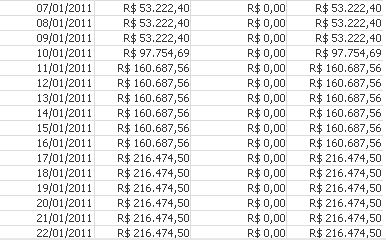# QlikView Scripting

Discussion Board for collaboration on QlikView Scripting.

Announcements
If you are a Qlik Insight Bot user, join this collaborative group: JOIN GROUP
New Contributor III

## Date of aggr formula

Fellows,

I have a formula to return maximum value exposed in a customer:

```Max(Aggr(Sum(If([Issue Date]<=Date,[Billed amount]))-Sum(If([Payment Date]<=Date,[Paid amount])),Date))
```

This sum all billed amount before a date and subtract all paid amount before the same date.

Aggr gives me an statement with balance (Billed amount - Paid amount) day after day (Date).

Columns are: Date, Billed amount, Paid amount, BalanceFirst objective was to get highest balance value. That is done.

Secondary is to get last Date when highest balance occurs (I am considering the possibility of having same balance for more than one row).

How to get this 'last Date'? I already tried Firstsortedvalue but not luck.

Tags (4)
1 Solution

Accepted Solutions
HighlightedMVP

## Re: Date of aggr formula

FirstSortedValue(Date,

-Aggr(Sum(If([Issue Date]<=Date,[Billed amount]))-Sum(If([Payment Date]<=Date,[Paid amount])),Date))

Or

FirstSortedValue(Distinct Date,

-Aggr(Sum(If([Issue Date]<=Date,[Billed amount]))-Sum(If([Payment Date]<=Date,[Paid amount])),Date))

5 Replies
HighlightedMVP

## Re: Date of aggr formula

FirstSortedValue(Date,

-Aggr(Sum(If([Issue Date]<=Date,[Billed amount]))-Sum(If([Payment Date]<=Date,[Paid amount])),Date))

Or

FirstSortedValue(Distinct Date,

-Aggr(Sum(If([Issue Date]<=Date,[Billed amount]))-Sum(If([Payment Date]<=Date,[Paid amount])),Date))

HighlightedMVP

## Re: Date of aggr formula

Would you be able to share your application or a sample with expected output?

HighlightedMVP

## Re: Date of aggr formula

FirstSortedValue(Distinct Date,

-Aggr(Sum(If([Issue Date]<=Date,[Billed amount]))-Sum(If([Payment Date]<=Date,[Paid amount]))-Date/1E6,Date))

Highlighted
New Contributor III

## Re: Date of aggr formula

Second option worked.

HighlightedMVP

## Re: Date of aggr formula

Just a note:

I don't think that

FirstSortedValue(Distinct Date,

-Aggr(Sum(If([Issue Date]<=Date,[Billed amount]))-Sum(If([Payment Date]<=Date,[Paid amount])),Date))

guarantees that the max date for the max balance is returned, it returns just any one date with the same sort weight, which one might be depending e.g. on the load order field Date.

If you want to make your expression more robust, follow e.g. Manish's last suggested solution (though I think the small value proportional to Date should be added, not subtracted, but could be wrong).